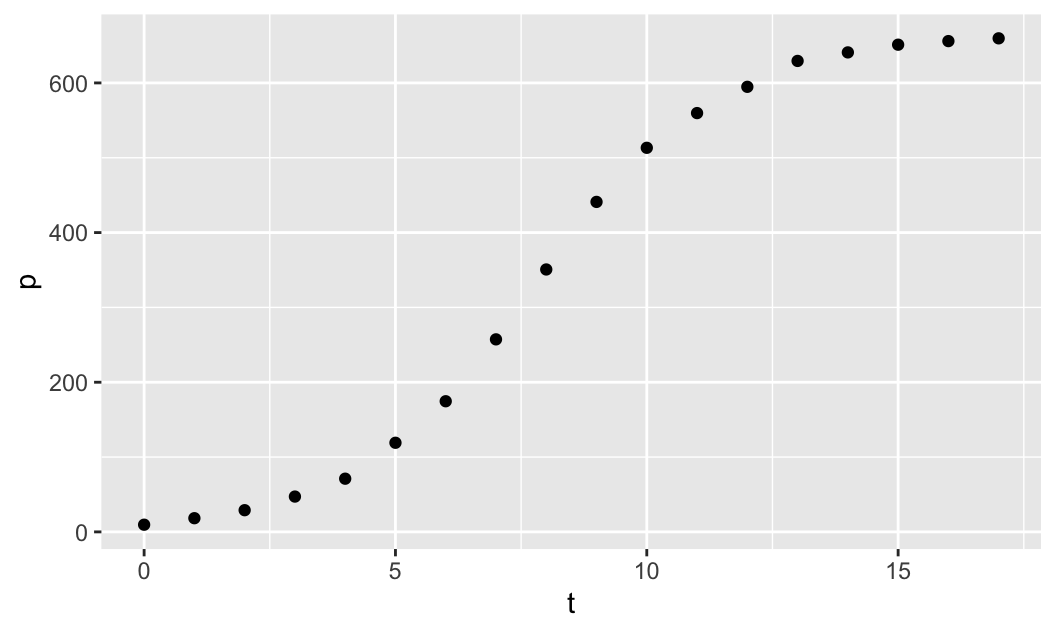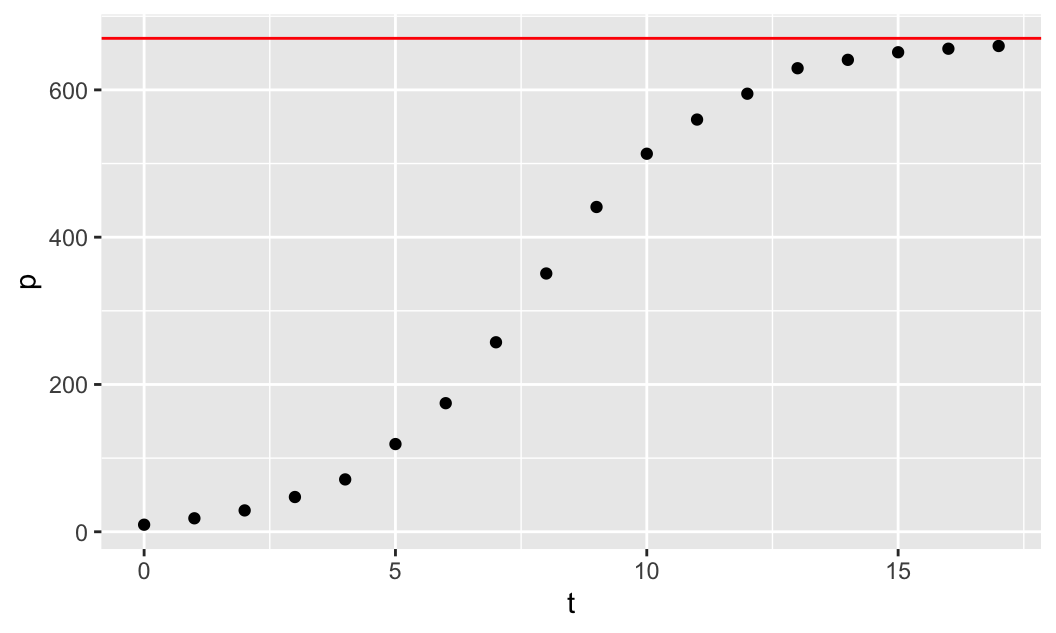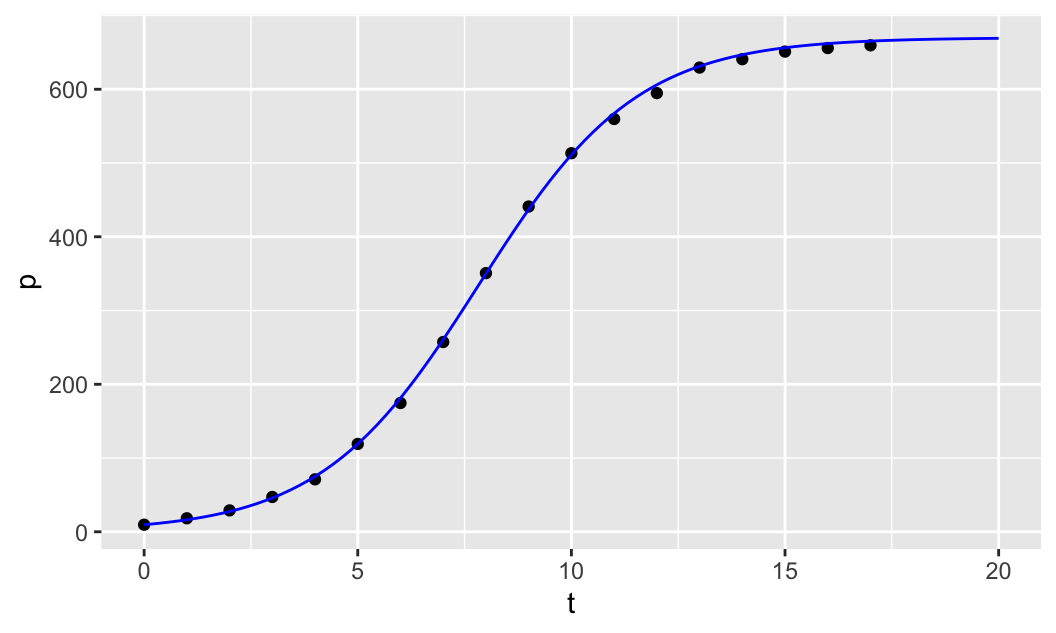# Chapter 1 Fitting a Logistic Model to Data

Example 1.1 We are given population data concerning the growth of a species of yeast in a closed vessel. Use the data given below and the solution $$u(t)$$ to the logistic differential equation to find estimates of the parameters $$u_0$$, $$r$$ and $$K$$.

$u(t) = \frac{K}{1+e^{-rt} \left (\frac{K}{u_0}-1 \right )}$ where $$K$$ is the carrying capacity of the population, $$r$$ is the growth rate and $$u_0$$ is the initial population size at time $$t=0$$. For more details, please review the lectures where we covered all details related to the solution of the logistic equation.

We use a graphical approach for this problem to develop some intuition about fitting the logistic model parameters to given data.

• What is $$u_0$$?
• What is a good estimate for $$K$$? What do you predict as the maximum sustainable population (carrying capacity), based on this model?
• What is a good estimate for $$r$$? Adjust the value of $$r$$ manually until the logistic solution fits the data well.
The yeast population p (in millions) as a function of time t (in hours), is given by the data below:
t<-0:17 # time in hours
# population in millions
p<-c(9.6, 18.3, 29.0, 47.2, 71.1, 119.1, 174.6, 257.3, 350.7,
441.0, 513.3, 559.7, 594.8, 629.4, 640.8, 651.1, 655.9, 659.6)

Hints:

• Start by creating a scatterplot of the data of yeast population against time.
• Find the horizontal asymptote to $$u(t)$$ by finding $$\lim_{t\to\infty}u(t)$$.
• Does the solution to the logistic equation level out? What is that level?
• Use the last observation above to make a good guess for the value of $$K$$.

Problem Solution:

# load the required packages
library(mosaic)
library(mosaicCalc)

First, we plot the population size p against time t, using the data given above. We use the plotting function gf_point(), which is available when you load the mosaic package to visualize the data. Note that the plotting function takes a formula p ~ t, where p and t are the given data vectors, and it creates the scatterplot of p against t:

gf_point(p ~ t)Next, we define the logistic solution as an R function u (the dependent variable) with argument t (the independent variable) using makeFun() from the mosaic package. Note that the R function u also depends on the parameters u0, r and K and it is created using the formula u(t) ~ t, which makes u a function of t:

u<-makeFun(K/(1+exp(-r*t)*(K/u0 - 1)) ~ t)

The initial yeast population at time zero is p=9.6, based on the data, so the parameter for the initial population is u0=9.6.

To find the horizontal asymptote, we need to find $$\lim_{t\to\infty}u(t)$$. As $$t\to\infty$$ the term $$e^{-rt}$$ goes to 0:

$\lim_{t\to\infty}u(t) = \lim_{t\to\infty}\frac{K}{1+0\cdot (K/u_0 -1)} = K$ Thus, the horizontal asymptote is $$u=K$$ (on the $$tu$$-plane). The yeast data appear to converge to the horizontal level of around 670, so we can take this as a reasonable estimate for $$K=670$$ to be the carrying capacity. We can plot the horizontal asymptote $$u=670$$ along with the data to make sure that it fits the data well:

gf_point(p ~ t) %>%
gf_hline(yintercept=670, col="red")In the code above, after we create the scatterplot of the data using gf_point(p ~ t), we then use the pipe operator %>%, which implements a composition of functions, and it composes the first plotting function with the second plotting function gf_hline, which plots the horizontal line $$u=670$$, for which we only need to specify the yintercept=670 (col="red" is optional).

Finally, to find a good estimate for $$r$$, we can manually adjust the value of $$r$$ until we get a good fit to the data. Note that we first create the scatter plot of the data as before, using gf_point(p ~ t), and then we use the pipe operator %>% to compose the two plotting functions. The second plotting function, composed with the first one, is the slice_plot() function from the mosaicCalc package. Note that slice_plot() takes the formula u(t,r=r, u0=u0, K=K) ~ t, where we give the parameters r, u0 and K specific numerical values and leave u to be a function of time t only. We then specify the domain over which to plot the graph of the function u(t) by giving a range for t, t=range(0,20) (col="blue" is optional).

u0=9.6 # initial population
K=670  # carrying capacity
r=0.54 # growth rate
gf_point(p ~ t) %>%
slice_plot(u(t,r=r, u0=u0, K=K) ~ t, domain(t=range(0,20)),
col="blue")We then start to manually adjust the parameter r until the model fits the data well.

Manually adjusting the parameter $$r$$ seems to produce a reasonable fit when $$r\approx 0.54$$.